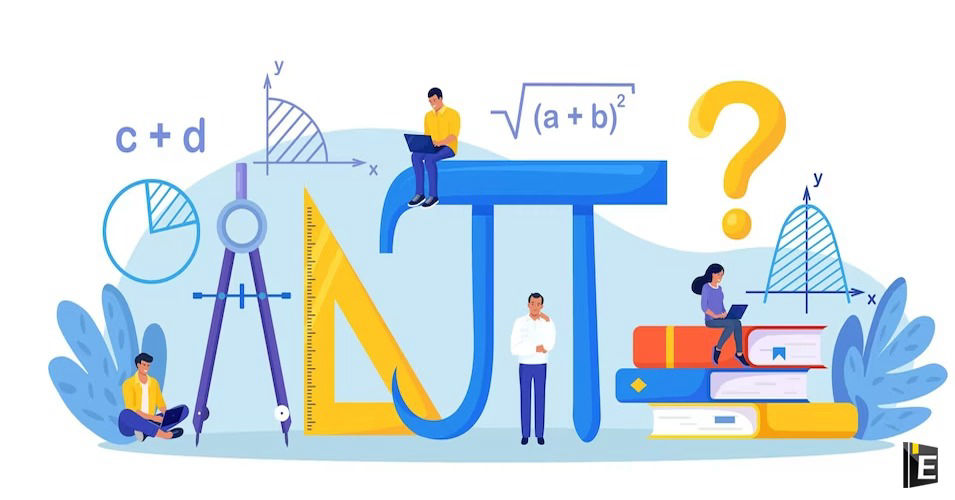• Save

# General Math Questions and Answers for SSC ExamGeneral Math Questions and Answers are an assessment that covers a wide range of mathematical concepts and topics. It typically consists of a series of questions that test one's knowledge of arithmetic, algebra, geometry, trigonometry, calculus, and other related subjects. General Math Questions and Answers can be used for educational purposes in schools and universities, or as a fun activity for individuals or groups interested in testing their math skills. They can be administered in person or online, and the difficulty level can vary depending on the target audience.

## General Math Questions

In this article, General Math Questions and Answers, you can get the latest and important questions related to Time and Distance, Time, Simple Interest, Simplification, HCF and LCM, Average, Decimal etc under the Aptitude and General Math section for upcoming competitive exams like SSC, RRB, BANK and other government exams.

Also, Read Latest Current Affairs Questions 2023: Current Affairs Today

Students can quickly get free General Knowledge Mock Test and Current Affairs Mock Test on this platform for online exam practice to obtain good marks in competitive exams.

## General Math Questions and Answers for SSC Exam

Q :

The average of 5 numbers, arranged in a row, is 309. The average of the first two of these numbers is 431 and the average of the last two of these numbers is 231.5. What is the value of the number at the center of the row?

(A) 321

(B) 52

(C) 108

(D) 220

Q :

What is the compound interest on ₹24,000 for 2 years at the rate of 10% per annum, if interest is compounded annually?

(A) ₹5,280

(B) ₹4,820

(C) ₹6,080

(D) ₹5,040

Q :

A boat takes 16 minutes and 24 minutes to cover a certain distance downstream and upstream, respectively. If the speed of the boat in still water is 15 km/h, find the speed of the stream.

(A) 2.5 km/h

(B) 3 km h

(C) 5 km/h

(D) 2 km/h

Q :

Two successive discounts of 20% and 30% are given while selling an article. What is the ratio of the marked price of the article to its selling price?

(A) 25:14

(B) 27:16

(C) 21:10

(D) 24:13

Q :

The average of five terms is 40. If the first four terms are given as 50, 52, 35 and 46, find the fifth term.

(A) 16

(B) 18

(C) 17

(D) 15

Q :

If the cost of 10 books is ₹250, then find the cost of 15 books.

(A) ₹125

(B) ₹115

(C) ₹375

(D) ₹215

Q :

Arun bought an old computer and spent ₹110 on its repairs. He then sold it to Bhola at a profit of 20%. Bhola sold it to Chandan at a loss of 10%. Chandan finally sold it for ₹1,188 at a profit of 10%. How much did Arun buy the computer for?

(A) ₹890

(B) ₹640

(C) ₹750

(D) ₹1,050

Q :

A cube has sides that are 4 cm each, and the dimensions of a cuboid are 2cm x 3cm x 4cm. Find the positive difference between the volume of the cube and that of the cuboid.

(A)

40 cm3

(B)

120 cm3

(C)

20 cm3

(D)

60 cm3

Q :

A goat is tied with a 10.5 m-long rope to one corner of a plot which is in the shape of an equilateral triangle each side of which is 16 m long. Find the area that the goat can graze on.

(A)

62.25 m2

(B)

57.75 m2

(C)

53.5 m2

(D)

51.75 m2

Q :

The ratio of the number of hens to the number of goats on farm A is 5: 4 and that on farm B is 5 : 7. What could be the ratio of the total number of hens to the total number of goats in farms A and B taken together?

(A) 3 : 2

(B) 7 : 11

(C) 1 : 2

(D) 9 : 11